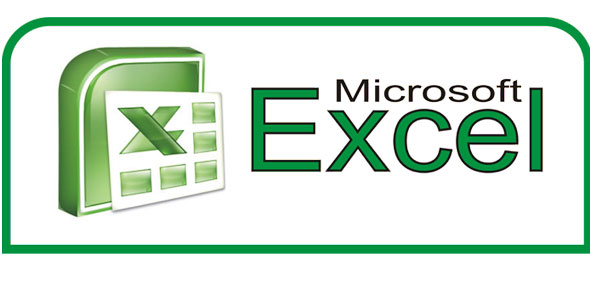# MS Excell Exam

25 Questions | Total Attempts: 102Settings.

Related Topics
• 1.
How do you change column width to fit the contents?
• A.

Single-click the boundary to the left of the column heading.

• B.

Double-click the boundary to the right of the column heading

• C.

Press ALT and single-click anywhere in the column

• D.

I don't know

• 2.
A fast way to add up this column of numbers is to click in the cell below the numbers and then:
• A.

Click Subtotals on the View menu

• B.

View the sum in the formula bar

• C.

Click the AutoSum button on the Home, then press ENTER

• D.

I don' t Know

• 3.
Assuming you are working in MS Excel and unfortunately, NEPA took the power and your work has not been saved. UPS is weak. What quickest way can you used to save your job before the UPS goes off?
• A.

Press Ctrl S

• B.

Off the UPS immediately without saving

• C.

Press Control X

• D.

• 4.
In order to save a new document in Microsoft Excel 2007, you must select which one of the following options?
• A.

Edit

• B.

Format

• C.

Help

• D.

Office Button

• 5.
What is the function of the word '=SUM' at the beginning of an Excel spreadsheet formula?
• A.

• B.

To tell the person viewing that this is a function and it should be added together

• C.

To calculate all the data correctly without any mistakes

• D.

To inform the computer that an arithmetic function will occur

• 6.
Which of the following INCORRECTLY selects multiple cells?
• A.

(A1:G50)

• B.

(A1, B3:C9)

• C.

(A1:B5:C5)

• D.

(A1:B5#C5)

• 7.
What do you type into an empty cell to start a formula?
• A.

Fx

• B.

An equal sign (=)

• C.

Number

• D.

• 8.
You can use Excel to create budgets, to work with taxes, or to record student grades
• A.

False

• B.

True

• C.

Not Sure

• D.

I dont know

• 9.
You learned in the practice that ###### means
• A.

You've entered a number wrong

• B.

You've misspelled something

• C.

The cell is not wide enough to contain its contents

• D.

Don't know

• 10.
You can enter two basic kinds of data into worksheet cells:
• A.

Numbers and text

• B.

Numbers and figures

• C.

None of the above

• D.

• 11.
A formula result is in cell C6. You wonder how you got the result. To see the formula, you:
• A.

Click in cell C6, and then press CTRL+SHIFT.

• B.

Click in cell C6.

• C.

Non of the above

• D.

Wait for Bro. Samson to show you

• 12.
___________ are where you get down to business and enter data in a worksheet
• A.

Cells

• B.

Fields

• C.

Tables

• D.

None of the above

• 13.
To divide 853 by 16 in a formula in Excel, you would use what math operator?
• A.

+

• B.

=

• C.

%

• D.

/

• 14.
_______, ________ and _______ are what you see when you open Excel
• A.

Columns, rows, and charts

• B.

Columns, rows, and formulas

• C.

Columns, rows, and cells

• D.

Non of the above

• 15.
Which function will you use to enter current date and time in a woksheet cell?
• A.

=today()

• B.

=now()

• C.

=time()

• D.

=currentTime()

• 16.
Formatting a cell in Currency, you can specify
• A.

Decimal Places

• B.

Currency Symbol

• C.

Both of above

• D.

None of above

• 17.
Which of the following is not an option in the spelling dialog?
• A.

Ignore

• B.

Ignore all

• C.

Change

• D.

I dont know

• 18.
Which one do you like?
• A.

Option 1

• B.

Option 2

• C.

Option 3

• D.

Option 4

• 19.
Which do you press to enter the current date in a cell?
• A.

CTRL+; (semicolon)

• B.

CTRL+SHIFT+: (colon)

• C.

CTRL+F10

• D.

I forgot

• 20.
A user wishes to remove a spreadsheet from a workbook. Which is the correct sequence of events that will do this?
• A.

Right click on the spreadsheet tab and select DELETE

• B.

Go to File-Save As – Save As Type – Excel worksheet

• C.

Right click on the spreadsheet and select Insert – Entire Column

• D.

Uncle Samson did not tell me

• 21.
When you open an Excel workbook, there are__________ sheets by default
• A.

Four

• B.

Three

• C.

Two

• D.

One

• 22.
Which of the cell pointer indicate that you can move the content to other cell?
• A.

Doctor’s symbol (Big Plus)

• B.

Small thin plus icon

• C.

Mouse Pointer with anchor at the tip

• D.

None of above

• 23.
To apply conditional formatting, you do the following except
• A.

Select the cells you would like to format

• B.

Select the color scales

• C.

Select Home tab

• D.

Locate the styles group

• 24.
Under which Excel Tab can we find "Format Painter"
• A.

Insert

• B.

Home

• C.

Review

• D.

View

• 25.
What Version of Ms Excel were you taught?
• A.

MS Excel 2000

• B.

MS Excel 2020

• C.

MS Excel 2007, 2010 and 2013

• D.

MS Excel Unnamed